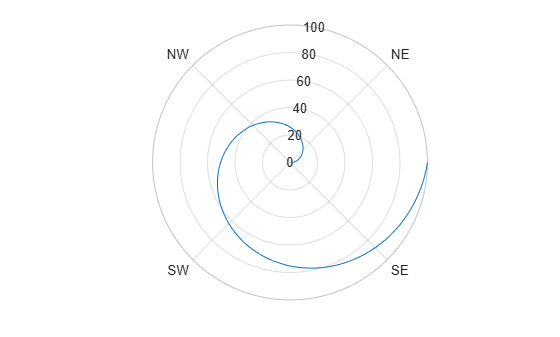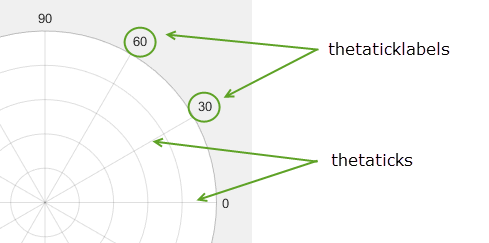# thetaticklabels

## 语法

thetaticklabels(labels)
tl = thetaticklabels
thetaticklabels('auto')
thetaticklabels('manual')
m = thetaticklabels('mode')
___ = thetaticklabels(pax,___)

## 说明

thetaticklabels(labels) 设置当前坐标区的 theta 轴刻度标签。可将 labels 指定为字符串数组或字符向量元胞数组，例如 {'E','N','W','S'}。如果指定标签，则 theta 轴刻度值和刻度标签不再基于坐标区的更改而自动更新。
tl = thetaticklabels 返回当前坐标区的 theta 轴刻度标签。

thetaticklabels('auto') 设置自动模式，使坐标区确定 theta 轴刻度标签。如果您设置了标签，然后又想将它们设置回默认值，则可以使用此选项。
thetaticklabels('manual') 设置手动模式，将 theta 轴刻度标签冻结在当前值。
m = thetaticklabels('mode') 返回 theta 轴刻度标签模式的当前值：'auto' 或 'manual'。默认情况下，该模式为自动，除非您指定刻度标签或将模式设置为手动。

___ = thetaticklabels(pax,___) 使用 pax 指定的坐标区，而不是使用当前坐标区。指定 ax 作为上述任何语法的第一个输入参数。

## 示例

theta = linspace(0,2*pi); rho = theta/10; polarplot(theta,rho) thetaticks([0 90 180 270]) thetaticklabels({'East','North','West','South'})theta = 0:0.01:2*pi; rho = 50*sin(2*theta); polarplot(theta,rho) pax = gca; thetaticks(pax,[0 90 180 270]) thetaticklabels(pax,{'East','North','West','South'})polarplot(1:100) thetaticks([45 135 225 315]) thetaticklabels({'NE','NW','SW','SE'})thetaticks('auto') thetaticklabels('auto')theta = 0:0.01:2*pi; rho = 50*sin(2*theta); polarplot(theta,rho) thetaticklabels({})## 输入参数

• 要指定刻度值，请使用 thetaticks 函数。

• 如果没有为所有刻度值指定足够的标签，则 MATLAB® 会为其余的刻度使用标签后跟空字符向量。

• 如果将刻度标签指定为分类数组，MATLAB 将使用数组中的值，而不是类别。

## 输出参数

• 'auto' - 坐标区自动确定 theta 轴刻度标签。

• 'manual' - 坐标区使用手动指定的 theta 轴刻度标签。

## 详细信息

### 刻度标签## 算法

thetaticklabels 函数设置并查询与 theta 轴刻度标签有关的多个极坐标区属性。

• ThetaTickLabel - 用于存储 theta 轴刻度标签的文本的属性。

• ThetaTickLabelMode - 用于存储 theta 轴刻度标签模式的属性。使用 thetaticklabels 设置 theta 轴刻度标签时，此属性将更改为 'manual'

• ThetaTickMode - 用于存储 theta 轴刻度值模式的属性。使用 thetaticklabels 设置 theta 轴刻度标签时，此属性将更改为 'manual'